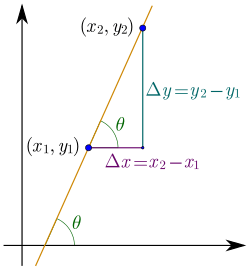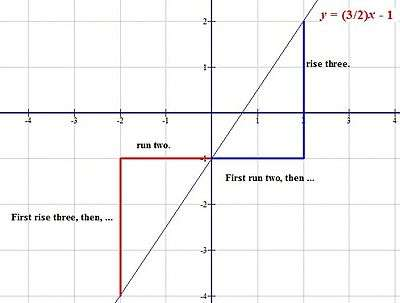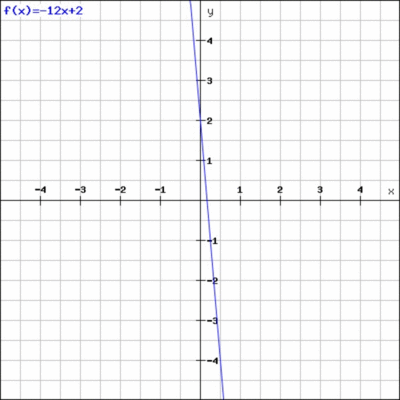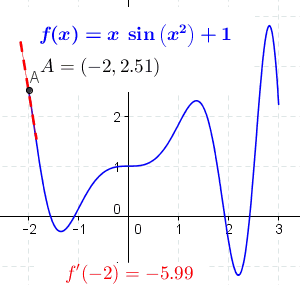# Slope

For the grade (incline or gradient or pitch or slope) of any physical feature, see Grade (slope).Slope:In mathematics, the slope or gradient of a line is a number that describes both the direction and the steepness of the line. Slope is often denoted by the letter m; there is no clear answer to the question why the letter m is used for slope, but it might be from the "m for multiple" in the equation of a straight line "y = mx + c".

• The direction of a line is either increasing, decreasing, horizontal or vertical.
• A line is increasing if it goes up from left to right. The slope is positive, i.e..
• A line is decreasing if it goes down from left to right. The slope is negative, i.e..
• If a line is horizontal the slope is zero. This is a constant function.
• If a line is vertical the slope is undefined (see below).
• The steepness, incline, or grade of a line is measured by the absolute value of the slope. A slope with a greater absolute value indicates a steeper line

Slope is calculated by finding the ratio of the "vertical change" to the "horizontal change" between (any) two distinct points on a line. Sometimes the ratio is expressed as a quotient ("rise over run"), giving the same number for every two distinct points on the same line. A line that is decreasing has a negative "rise". The line may be practical - as set by a road surveyor, or in a diagram that models a road or a roof either as a description or as a plan.

The rise of a road between two points is the difference between the altitude of the road at those two points, say y1 and y2, or in other words, the rise is (y2y1) = Δy. For relatively short distances - where the earth's curvature may be neglected, the run is the difference in distance from a fixed point measured along a level, horizontal line, or in other words, the run is (x2x1) = Δx. Here the slope of the road between the two points is simply described as the ratio of the altitude change to the horizontal distance between any two points on the line.

In mathematical language, the slope m of the line isThe concept of slope applies directly to grades or gradients in geography and civil engineering. Through trigonometry, the grade m of a road is related to its angle of incline θ by the tangent functionThus, a 45° rising line has a slope of +1 and a 45° falling line has a slope of 1.

As a generalization of this practical description, the mathematics of differential calculus defines the slope of a curve at a point as the slope of the tangent line at that point. When the curve given by a series of points in a diagram or in a list of the coordinates of points, the slope may be calculated not at a point but between any two given points. When the curve is given as a continuous function, perhaps as an algebraic formula, then the differential calculus provides rules giving a formula for the slope of the curve at any point in the middle of the curve.

This generalization of the concept of slope allows very complex constructions to be planned and built that go well beyond static structures that are either horizontals or verticals, but can change in time, move in curves, and change depending on the rate of change of other factors. Thereby, the simple idea of slope becomes one of the main basis of the modern world in terms of both technology and the built environment.

## DefinitionSlope illustrated for y = (3/2)x  1. Click on to enlargeSlope of a line in coordinates system, from f(x)=-12x+2 to f(x)=12x+2

The slope of a line in the plane containing the x and y axes is generally represented by the letter m, and is defined as the change in the y coordinate divided by the corresponding change in the x coordinate, between two distinct points on the line. This is described by the following equation:(The Greek letter delta, Δ, is commonly used in mathematics to mean "difference" or "change".)

Given two points (x1,y1) and (x2,y2), the change in x from one to the other is x2x1 (run), while the change in y is y2y1 (rise). Substituting both quantities into the above equation generates the formula:The formula fails for a vertical line, parallel to the y axis (see Division by zero), where the slope can be taken as infinite, so the slope of a vertical line is considered undefined.

### Examples

Suppose a line runs through two points: P = (1, 2) and Q = (13, 8). By dividing the difference in y-coordinates by the difference in x-coordinates, one can obtain the slope of the line:.
Since the slope is positive, the direction of the line is increasing. Since |m|<1, the incline is not very steep (incline <45°).

As another example, consider a line which runs through the points (4, 15) and (3, 21). Then, the slope of the line isSince the slope is negative, the direction of the line is decreasing. Since |m|>1, this decline is fairly steep (decline >45°).

## Algebra and geometry

• If y is a linear function of x, then the coefficient of x is the slope of the line created by plotting the function. Therefore, if the equation of the line is given in the formthen m is the slope. This form of a line's equation is called the slope-intercept form, because c can be interpreted as the y-intercept of the line, that is, the y-coordinate where the line intersects the y-axis.
• If the slope m of a line and a point (x1,y1) on the line are both known, then the equation of the line can be found using the point-slope formula:is.
• Two lines are parallel if and only if they are not the same line (coincident) and either their slopes are equal or they both are vertical and therefore both have undefined slopes. Two lines are perpendicular if the product of their slopes is 1 or one has a slope of 0 (a horizontal line) and the other has an undefined slope (a vertical line).
• The angle θ between -90° and 90° that a line makes with the x-axis is related to the slope m as follows:and(this is the inverse function of tangent; see trigonometry).

### Examples

For example, consider a line running through the points (2,8) and (3,20). This line has a slope, m, ofOne can then write the line's equation, in point-slope form:or:The angle θ between -90° and 90° that this line makes with the x axis isConsider the two lines: y = -3x + 1 and y = -3 x - 2. Both lines have slope m = -3. They are not the same line. So they are parallel lines.

Consider the two lines y = -3x + 1 and y = x/3 - 2. The slope of the first line is m1 = -3. The slope of the second line is m2 = 1/3. The product of these two slopes is -1. So these two lines are perpendicular.

## Slope of a road or railway

There are two common ways to describe the steepness of a road or railroad. One is by the angle between 0° and 90° (in degrees), and the other is by the slope in a percentage. See also steep grade railway and rack railway.

The formulae for converting a slope given as a percentage into an angle in degrees and vice versa are:, (this is the inverse function of tangent; see trigonometry)
andwhere angle is in degrees and the trigonometric functions operate in degrees. For example, a slope of 100% or 1000 is an angle of 45°.

A third way is to give one unit of rise in say 10, 20, 50 or 100 horizontal units, e.g. 1:10. 1:20, 1:50 or 1:100 (or "1 in 10", "1 in 20" etc.) Note that 1:10 is steeper than 1:20. For example, steepness of 20% means 1:5 or an incline with angle 11,3°.

Roads and railways have both longitudinal slopes and cross slopes.

## CalculusThe concept of a slope is central to differential calculus. For non-linear functions, the rate of change varies along the curve. The derivative of the function at a point is the slope of the line tangent to the curve at the point, and is thus equal to the rate of change of the function at that point.

If we let Δx and Δy be the distances (along the x and y axes, respectively) between two points on a curve, then the slope given by the above definition,,

is the slope of a secant line to the curve. For a line, the secant between any two points is the line itself, but this is not the case for any other type of curve.

For example, the slope of the secant intersecting y = x2 at (0,0) and (3,9) is 3. (The slope of the tangent at x = 32 is also 3—a consequence of the mean value theorem.)

By moving the two points closer together so that Δy and Δx decrease, the secant line more closely approximates a tangent line to the curve, and as such the slope of the secant approaches that of the tangent. Using differential calculus, we can determine the limit, or the value that Δyx approaches as Δy and Δx get closer to zero; it follows that this limit is the exact slope of the tangent. If y is dependent on x, then it is sufficient to take the limit where only Δx approaches zero. Therefore, the slope of the tangent is the limit of Δyx as Δx approaches zero, or dy/dx. We call this limit the derivative.Its value at a point on the function gives us the slope of the tangent at that point. For example, let y=x2. A point on this function is (-2,4). The derivative of this function is dy/dx=2x. So the slope of the line tangent to y at (-2,4) is 2·(-2) = -4. The equation of this tangent line is: y-4=(-4)(x-(-2)) or y = -4x - 4.

## Other generalizations

The concept of slope can be generalized to functions of more than one variable and is more often referred to as gradient.Look up slope in Wiktionary, the free dictionary.